# Approximation of solutions of Hammerstein equations with monotone mappings in real Banach spaces

### Abstractcarpathian_2019_35_3_305_316_abstract

Issue no:

Let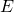be a uniformly convex and uniformly smooth real Banach space with dual space,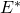. Let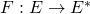,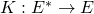be maximal monotone mappings. An iterative algorithm is constructed and the sequence of the algorithm is proved to converge strongly to a solution of the Hammerstein equation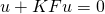. This theorem is a significant improvement of some important recent results which were proved in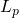spaces,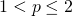under the assumption that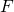and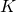are  bounded. This restriction onandhave been dispensed with even in the more general setting considered here. Finally, a numerical experiment is  presented to illustrate the convergence of the sequence of the algorithm which is found to be much faster, in terms of the number of  iterations and the computational time than the convergence obtained with existing algorithms.